# Introduction to Exploring Data in Python¶

In this lesson, we're going to learn about how to get a feel for data in Python, using basic tools to look at our data.

First, however, let's make sure we have the right version of a library we need called Seaborn. Run the code in the next block and make sure that you get the right version.

In :
import warnings
warnings.filterwarnings("ignore")

import seaborn as sns
sns.__version__

Out:
'0.9.0'

Make sure that you have version 0.9.0 or higher as the output. If you don't, the code in this lesson will not work.

If you have a lower version (you might have 0.8.0) then start a new cell and run the following code:

!pip install seaborn --upgrade

Then, when that finishes installing (the asterisk goes away), delete that cell and select kernel -> restart and run all from the menus up above. If everything worked ok, it should now say 0.9.0 or higher. If not, consult with me.

We're also going to import Pandas, which is a very powerful library for reading and accessing tabular data (like spreadsheets); you can think of Pandas as souped-up Excel for programmers. We will also import the built in io library and the Requests library for making requests from the internet; we'll see why in a moment.

In :
import pandas as pd
import io, requests


This next block is a little gnarly. What we're going to do is use the get() function in the requests library to fetch a good learning dataset from another professor's class this class, if you're curious, and then turn it into a Pandas DataFrame (fancy spreadsheet). There's a bit of messiness that you don't quite need to worry about in this---particularly, read_fwf() is a pandas function to read from a file of fixed-width lines, and StringIO is how Python tricks libraries to think that a string (the result of our http request) is a file.

For an exercise, go and look up the Pandas documentation for how you'd read a CSV file, then go find a CSV file online and load it up to a Pandas dataset yourself.

In :
raw_data = requests.get("http://data.princeton.edu/wws509/datasets/salary.dat").text

In :
df.head()

Out:
sx rk yr dg yd sl
0 male full 25 doctorate 35 36350
1 male full 13 doctorate 22 35350
2 male full 10 doctorate 23 28200
3 female full 7 doctorate 27 26775
4 male full 19 masters 30 33696

We just used the head() method on our DataFrame, df, to see the first few rows of the data. This is a great way to get a quick look at what the variables are in a dataset, what type they are, and so forth.

(Note: the formatting of these tables on the course website might not be amazing. I'm working on it, and if you see this message, I may not yet have fixed it. You can always look in the course github repository for lessons too, where the formatting is a bit cleaner.)

One immediately annoying thing about this dataset is that the variables have terrible names. Let's fix that.

In :
df.columns=["sex", "rank", "years_in_rank", "highest_degree", "years_since_degree", "salary"]

In :
df.head()

Out:
sex rank years_in_rank highest_degree years_since_degree salary
0 male full 25 doctorate 35 36350
1 male full 13 doctorate 22 35350
2 male full 10 doctorate 23 28200
3 female full 7 doctorate 27 26775
4 male full 19 masters 30 33696

We can also learn a lot about a DataFrame by using the describe() and value_counts() methods.

In :
df.describe()

Out:
years_in_rank years_since_degree salary
count 52.000000 52.000000 52.000000
mean 7.480769 16.115385 23797.653846
std 5.507536 10.222340 5917.289154
min 0.000000 1.000000 15000.000000
25% 3.000000 6.750000 18246.750000
50% 7.000000 15.500000 23719.000000
75% 11.000000 23.250000 27258.500000
max 25.000000 35.000000 38045.000000
In :
df.sex.value_counts('')

Out:
male      38
female    14
Name: sex, dtype: int64

So, obviously, this is a dataset about a theoretical gender discrimination in university employment problem, and one obvious thing we might want to know here is whether there's a gender disparity by rank: are higher ranking faculty in our dataset more likely to be one gender or another?

One classic exploratory tool to get a sense of whether this is true is known as a crosstab. Basically, that's just a fancy name for a table---we might want to know how many assistant professors there are of each gender, associate professors, etc. That's pretty easy to do in Pandas.

This kind of table is also known as a contingency table.

In :
pd.crosstab(index=df['sex'], columns=df['rank'], margins=True)

Out:
rank assistant associate full All
sex
female 8 2 4 14
male 10 12 16 38
All 18 14 20 52

We can see right away that there is a gender disparity. More than half of women are at the assistant (lowest) rank, while less than a third of men are there; men are more likely to be full professors (the highest rank) than anything else. Note that this isn't evidence of discrimination---after all, there might be some other reason for this disparity (and if this pattern didn't show up, it wouldn't be evidence of no discrimination). To get serious evidence, we'll have to look at some of the things that we'll talk about in the statistics part of the course. Nonetheless, it's always good to get a basic look at your data first.

Another great way to understand what your data is all about is to use visualizations. For this, we'll make use of the Seaborn library that we loaded up right at the beginning of this lesson.

The first visualization tool in our kit is the histogram. This allows us to visualize the distribution of a single variable in our data---to see how much of our data takes different kinds of values. Let's look at a histogram of the salary data in this set. In Seaborn, we do this with the distplot() function (for reasons that are a bit obscure---almost every other library calls it "hist").

In :
sns.distplot(df["salary"])

Out:
<matplotlib.axes._subplots.AxesSubplot at 0x118766b00>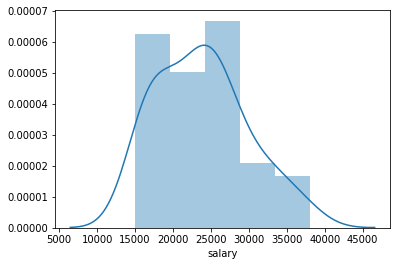This allows us to see the rough shape of our data. There are a bunch of salaries, it looks like, between 15,000 and 30,000-ish, and many fewer salaries above that. (Ignore the line drawn through this plot for now, that's a fancy thing called a "kernel density estimate")

You should always start by looking at histograms of your variables of interest.

One gotcha with histograms is that the bin size makes a big difference. What a histogram does is chunks our data into groupings, each with the same size, and then makes a bar chart of those groups. Seaborn has an algorithm that by default usually makes a pretty sensible selection, but it's always good to look at different bin widths---it can make a big difference.

In the existing data, it looks like the default Seaborn algorithm chose 5 bins, which seems sensible, but let's change that and see what happens!

In :
sns.distplot(df["salary"], bins=10)

Out:
<matplotlib.axes._subplots.AxesSubplot at 0x1188680f0>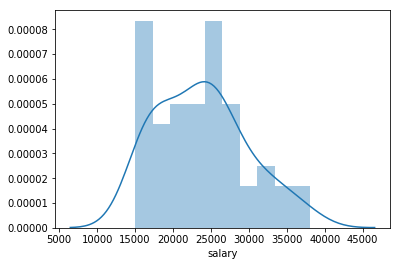In :
sns.distplot(df["salary"], bins=20)

Out:
<matplotlib.axes._subplots.AxesSubplot at 0x1187654e0>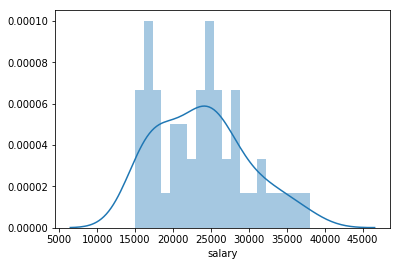In :
sns.distplot(df["salary"], bins=3)

Out:
<matplotlib.axes._subplots.AxesSubplot at 0x118a5bb70>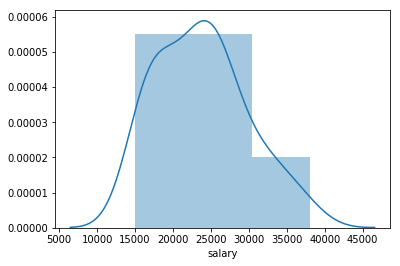In :
sns.distplot(df["salary"], bins=2)

Out:
<matplotlib.axes._subplots.AxesSubplot at 0x118b2afd0>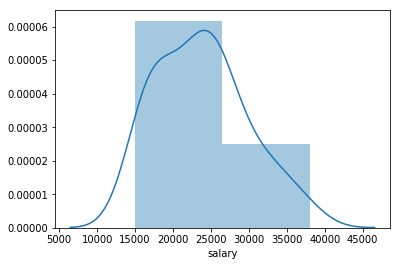Depending on how gnarly your dataset is, you might see very different things at different numbers of bins. So you should look at many.

Another classic standby is the scatterplot which is how we get a first look at how two different numerical variables relate to one another. Let's see how years since degree relate to salary.

In :
sns.scatterplot(x=df["years_since_degree"], y=df["salary"])

Out:
<matplotlib.axes._subplots.AxesSubplot at 0x118bfb550>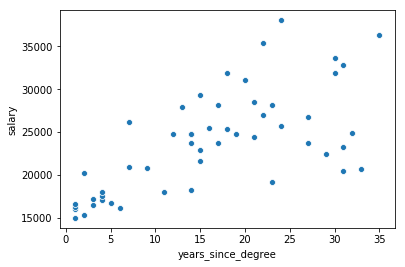In a scatterplot, each observation is a dot, and the location of a dot is determined by its value on the two variables. So it looks like on the whole, the further out from degree someone is, the higher their salary is. This is as to be expected---obviously, more experienced people get paid more.

## Discrete vs Continuous variables¶

One thing that has been in the background of our lesson thus far is the difference between categorical data, also known as discrete data, and numerical data, also known as "continuous" data.

Discrete data is, loosely speaking, data that takes a fixed number of values in your dataset. For example, in the dataset we're working with, gender only takes female and male, it's a discrete variable. Income, by contrast, is continuous because within its range, it could be anything. (Of course, strictly speaking, this isn't true, because you can't pay people fractions of a penny, and in our data we only have whole dollars. But it's close enough---these categories are kind of rough.) You could make salary discrete by putting it in bins --- if we created categories like "under 20,000", "20,000-25,000" and so forth, we could discretize that data.

This matters because different visualizations are more or less appropriate for different kinds of data. For example, scatterplots where one of the variables is discrete tend to be pretty useless. Take a look:

In :
sns.scatterplot(x=df["sex"], y=df["salary"])

Out:
<matplotlib.axes._subplots.AxesSubplot at 0x118cd52b0>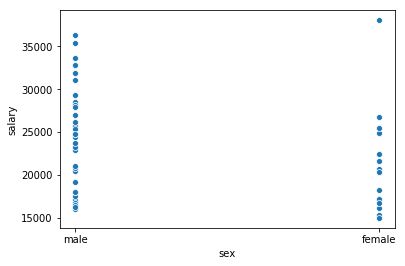That tells us something, but it doesn't tell us a whole lot! The reason is because there just isn't enough variation in the sex variable to let us see meaningful associations with this plot.

So what do you do then? Well, one neat thing we can do is use color to add a categorical dimensions to a scatterplot. Check this out:

In :
sns.scatterplot(x=df["years_since_degree"], y=df["salary"], hue=df["sex"])

Out:
<matplotlib.axes._subplots.AxesSubplot at 0x118d7fc50>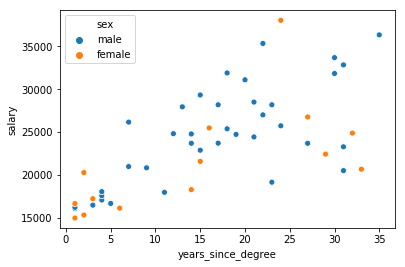That let us look at each datapoint and see the gender label attached to it! So we can see, for example, that it sort of looks like women are more or less concentrated below men, that is, that at the same number of years since degree, it sort of looks like women tend to be paid less. (We'll make "sort of looks like" much more precise when we learn about regression.)

Another good classic plot to understand how values of a numeric variable differ by a categorical variable is the boxplot:

In :
sns.boxplot(x=df["sex"], y=df["salary"])

Out:
<matplotlib.axes._subplots.AxesSubplot at 0x118e216a0>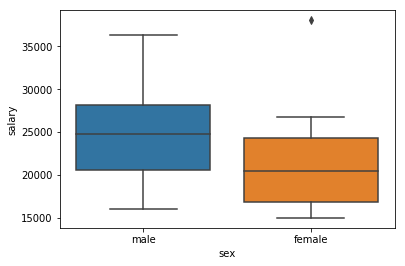The way to read a boxplot is that the boxes shows the interquartile range, or the middle 50% (i.e., the 25th percentile to the 75th percentile) of the distributions---in this case, of the distributions of salary by gender. The whiskers (bars) at the ends show the full range of the dataset, except for extreme values ("outliers"), which are shown in dots above and below. The bars in the middle of the boxes represent the mean (average).

Here, we can see that salaries for women, on the whole, tend to clump lower than men, except for one woman who is getting a much higher salary than everyone else.

The histogram, scatterplot, and boxplot are the Big Three for data visualizations---between the three of them and their variations, you can usually get a pretty good feel for the overall shape of your data, before you drill down and do analysis on them.

Let's look at a couple more fancy things. First, you can squeeze an extra color dimension in boxplots too, and that can help you see things like whether, for example, there's variation in salary by gender across different ranks:

In :
sns.boxplot(x=df["rank"], y=df["salary"], hue=df["sex"])

Out:
<matplotlib.axes._subplots.AxesSubplot at 0x118f2e550>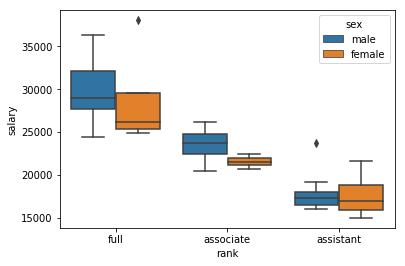The interpretation of that table should be pretty obvious In lots of ways, this looks worse for the gender equity in this notional department: it looks like higher-ranking women are getting distinctively less well paid than men, with the exeption of that one super-high-paid woman.

Another fancy plot is a violin plot, which is like a boxplot, except that it uses one of those kernel estimators to make it possible to visualize the rough number of data points in a given point on the y-axis scale, and doesn't do the interquartile range and outliers thing. Here's an example:

In :
sns.violinplot(x=df["rank"], y=df["salary"], hue=df["sex"], inner="stick")

Out:
<matplotlib.axes._subplots.AxesSubplot at 0x119056ac8>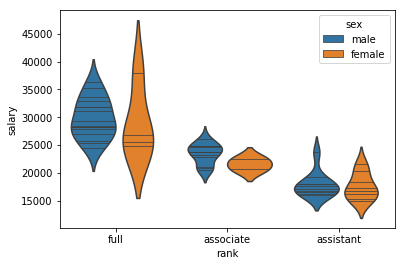As you can see, these look quite different from the boxplots, although they capture the same information. Note that I used an option to draw lines in the middle of each plot for each datapoint (that was the inner="stick" bit). There are lots of different options with violin plots, and the intuitions they give you can be a bit sensitive to how you choose those options, so I don't recommend them as a first choice. For example, this plot might make you think different things about the data:

In :
sns.violinplot(x=df["rank"], y=df["salary"], hue=df["sex"],  scale="count", inner="stick", scale_hue=False)

Out:
<matplotlib.axes._subplots.AxesSubplot at 0x119189128>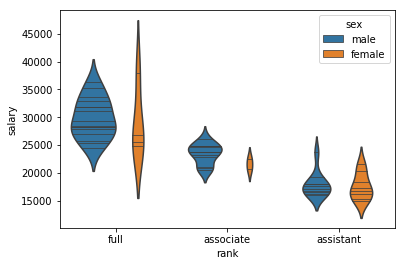What I did there was scale the sizes of the violins to the number of points in them. This is probably a bit more honest than the previous one, in terms of seeing where things actually lie.

For our last plot, let's fancy-up a boxplot some too by overlaying the actual points on it. This packs a ton of information in---I just want to show you what we can do with fancy plotting:

In :
fancyplot = sns.boxplot(x=df["rank"], y=df["salary"], hue=df["sex"], palette="pastel")
fancyplot = sns.swarmplot(x=df["rank"], y=df["salary"], hue=df["sex"], dodge=True, palette="dark")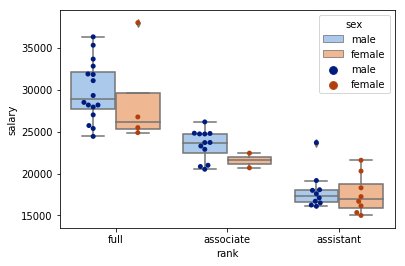I encourage you to look at the Seaborn tutorial for more examples of the cool stuff you can do with this library.